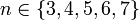# Classification of alternating groups that are N-groups

This article classifies the members in a particular group family alternating group that satisfy the group property N-group.

## Statement

Suppose$n$ is a positive integer greater than or equal to 3. The alternating group$A_n$ is a N-group if and only if$n \in \{ 3,4,5,6,7 \}$.

Similarly, the finitary alternating group on an infinite set is never a N-group.

## Proof

### Proof of failure for larger$n$

For$n \ge 8$, denote by$A_n$ the alternating group on the set$\{ 1,2,3,\dots,n\}$. Let$x = (1,2,3)$. The normalizer$N_G(\langle x \rangle)$ contains the centralizer of$x$, which in turn contains the alternating group on the set$\{4,5,\dots,n\}$, which is isomorphic to$A_{n-3}$, which is simple non-abelian since$n \ge 8 \implies n - 3 \ge 5$ (see alternating groups are simple). Thus,$N_G(\langle x \rangle)$ is not solvable, so$A_n$ is not a N-group.